This book is archived and will be removed July 6, 2022. Please use the updated version.

Inductance

# 94 Self-Inductance and Inductors

### Learning Objectives

By the end of this section, you will be able to:

• Correlate the rate of change of current to the induced emf created by that current in the same circuit
• Derive the self-inductance for a cylindrical solenoid
• Derive the self-inductance for a rectangular toroid

Mutual inductance arises when a current in one circuit produces a changing magnetic field that induces an emf in another circuit. But can the magnetic field affect the current in the original circuit that produced the field? The answer is yes, and this is the phenomenon called self-inductance.

### Inductors

(Figure) shows some of the magnetic field lines due to the current in a circular loop of wire. If the current is constant, the magnetic flux through the loop is also constant. However, if the current I were to vary with time—say, immediately after switch S is closed—then the magnetic fluxwould correspondingly change. Then Faraday’s law tells us that an emfwould be induced in the circuit, where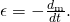Since the magnetic field due to a current-carrying wire is directly proportional to the current, the flux due to this field is also proportional to the current; that is,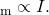A magnetic field is produced by the current I in the loop. If I were to vary with time, the magnetic flux through the loop would also vary and an emf would be induced in the loop.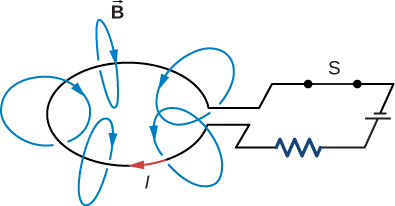This can also be written as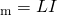where the constant of proportionality L is known as the self-inductance of the wire loop. If the loop has N turns, this equation becomes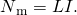By convention, the positive sense of the normal to the loop is related to the current by the right-hand rule, so in (Figure), the normal points downward. With this convention,is positive in (Figure), so L always has a positive value.

For a loop with N turns,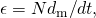so the induced emf may be written in terms of the self-inductance as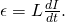When using this equation to determine L, it is easiest to ignore the signs of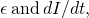and calculate L as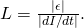Since self-inductance is associated with the magnetic field produced by a current, any configuration of conductors possesses self-inductance. For example, besides the wire loop, a long, straight wire has self-inductance, as does a coaxial cable. A coaxial cable is most commonly used by the cable television industry and may also be found connecting to your cable modem. Coaxial cables are used due to their ability to transmit electrical signals with minimal distortions. Coaxial cables have two long cylindrical conductors that possess current and a self-inductance that may have undesirable effects.

A circuit element used to provide self-inductance is known as an inductor. It is represented by the symbol shown in (Figure), which resembles a coil of wire, the basic form of the inductor. (Figure) shows several types of inductors commonly used in circuits.

Symbol used to represent an inductor in a circuit.A variety of inductors. Whether they are encapsulated like the top three shown or wound around in a coil like the bottom-most one, each is simply a relatively long coil of wire. (credit: Windell Oskay)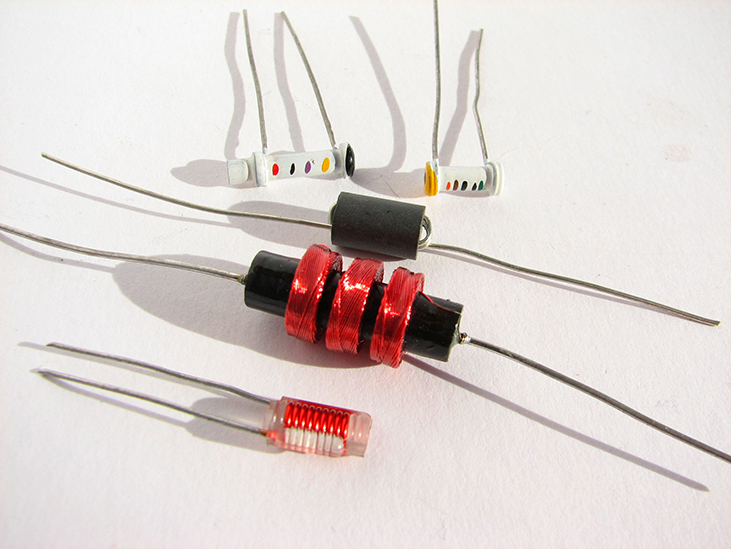In accordance with Lenz’s law, the negative sign in (Figure) indicates that the induced emf across an inductor always has a polarity that opposes the change in the current. For example, if the current flowing from A to B in (Figure)(a) were increasing, the induced emf (represented by the imaginary battery) would have the polarity shown in order to oppose the increase. If the current from A to B were decreasing, then the induced emf would have the opposite polarity, again to oppose the change in current ((Figure)(b)). Finally, if the current through the inductor were constant, no emf would be induced in the coil.

The induced emf across an inductor always acts to oppose the change in the current. This can be visualized as an imaginary battery causing current to flow to oppose the change in (a) and reinforce the change in (b).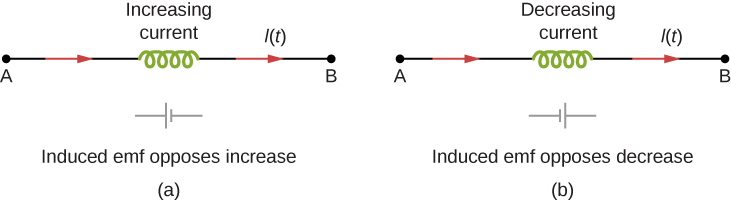One common application of inductance is to allow traffic signals to sense when vehicles are waiting at a street intersection. An electrical circuit with an inductor is placed in the road underneath the location where a waiting car will stop. The body of the car increases the inductance and the circuit changes, sending a signal to the traffic lights to change colors. Similarly, metal detectors used for airport security employ the same technique. A coil or inductor in the metal detector frame acts as both a transmitter and a receiver. The pulsed signal from the transmitter coil induces a signal in the receiver. The self-inductance of the circuit is affected by any metal object in the path ((Figure)). Metal detectors can be adjusted for sensitivity and can also sense the presence of metal on a person.

The familiar security gate at an airport not only detects metals, but can also indicate their approximate height above the floor. (credit: “Alexbuirds”/Wikimedia Commons)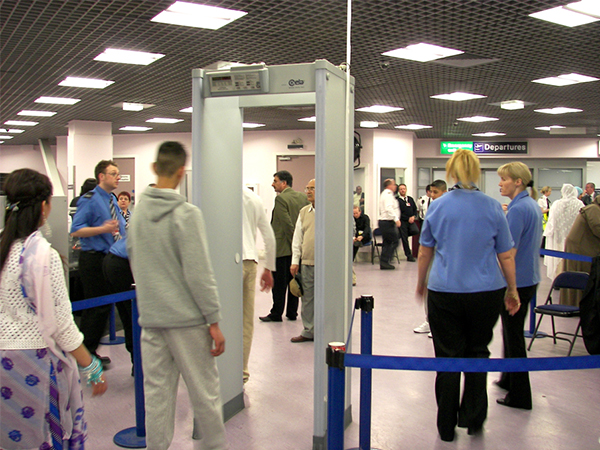Large induced voltages are found in camera flashes. Camera flashes use a battery, two inductors that function as a transformer, and a switching system or oscillator to induce large voltages. Recall from Oscillations on oscillations that “oscillation” is defined as the fluctuation of a quantity, or repeated regular fluctuations of a quantity, between two extreme values around an average value. Also recall (from Electromagnetic Induction on electromagnetic induction) that we need a changing magnetic field, brought about by a changing current, to induce a voltage in another coil. The oscillator system does this many times as the battery voltage is boosted to over 1000 volts. (You may hear the high-pitched whine from the transformer as the capacitor is being charged.) A capacitor stores the high voltage for later use in powering the flash.

Self-Inductance of a Coil An induced emf of 2.0 V is measured across a coil of 50 closely wound turns while the current through it increases uniformly from 0.0 to 5.0 A in 0.10 s. (a) What is the self-inductance of the coil? (b) With the current at 5.0 A, what is the flux through each turn of the coil?

Strategy Both parts of this problem give all the information needed to solve for the self-inductance in part (a) or the flux through each turn of the coil in part (b). The equations needed are (Figure) for part (a) and (Figure) for part (b).

Solution

1. Ignoring the negative sign and using magnitudes, we have, from (Figure),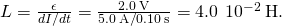2. From (Figure), the flux is given in terms of the current by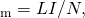so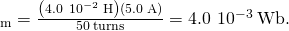Significance The self-inductance and flux calculated in parts (a) and (b) are typical values for coils found in contemporary devices. If the current is not changing over time, the flux is not changing in time, so no emf is induced.

Check Your Understanding Current flows through the inductor in (Figure) from B to A instead of from A to B as shown. Is the current increasing or decreasing in order to produce the emf given in diagram (a)? In diagram (b)?

a. decreasing; b. increasing; Since the current flows in the opposite direction of the diagram, in order to get a positive emf on the left-hand side of diagram (a), we need to decrease the current to the left, which creates a reinforced emf where the positive end is on the left-hand side. To get a positive emf on the right-hand side of diagram (b), we need to increase the current to the left, which creates a reinforced emf where the positive end is on the right-hand side.

Check Your Understanding A changing current induces an emf of 10 V across a 0.25-H inductor. What is the rate at which the current is changing?

40 A/s

A good approach for calculating the self-inductance of an inductor consists of the following steps:

Problem-Solving Strategy: Self-Inductance
1. Assume a current I is flowing through the inductor.
2. Determine the magnetic field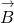produced by the current. If there is appropriate symmetry, you may be able to do this with Ampère’s law.
3. Obtain the magnetic flux,4. With the flux known, the self-inductance can be found from (Figure),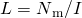.

To demonstrate this procedure, we now calculate the self-inductances of two inductors.

### Cylindrical Solenoid

Consider a long, cylindrical solenoid with length l, cross-sectional area A, and N turns of wire. We assume that the length of the solenoid is so much larger than its diameter that we can take the magnetic field to be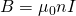throughout the interior of the solenoid, that is, we ignore end effects in the solenoid. With a current I flowing through the coils, the magnetic field produced within the solenoid is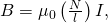so the magnetic flux through one turn is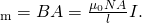Using (Figure), we find for the self-inductance of the solenoid,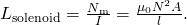If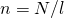is the number of turns per unit length of the solenoid, we may write (Figure) aswhere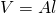is the volume of the solenoid. Notice that the self-inductance of a long solenoid depends only on its physical properties (such as the number of turns of wire per unit length and the volume), and not on the magnetic field or the current. This is true for inductors in general.

### Rectangular Toroid

A toroid with a rectangular cross-section is shown in (Figure). The inner and outer radii of the toroid are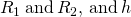is the height of the toroid. Applying Ampère’s law in the same manner as we did in (Figure) for a toroid with a circular cross-section, we find the magnetic field inside a rectangular toroid is also given by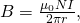where r is the distance from the central axis of the toroid. Because the field changes within the toroid, we must calculate the flux by integrating over the toroid’s cross-section. Using the infinitesimal cross-sectional area element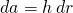shown in (Figure), we obtain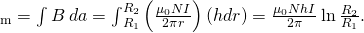Calculating the self-inductance of a rectangular toroid.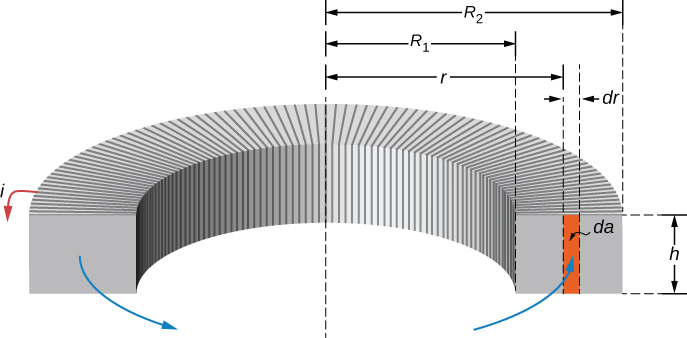Now from (Figure), we obtain for the self-inductance of a rectangular toroid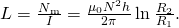As expected, the self-inductance is a constant determined by only the physical properties of the toroid.

Check Your Understanding (a) Calculate the self-inductance of a solenoid that is tightly wound with wire of diameter 0.10 cm, has a cross-sectional area of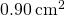, and is 40 cm long. (b) If the current through the solenoid decreases uniformly from 10 to 0 A in 0.10 s, what is the emf induced between the ends of the solenoid?

a.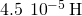; b.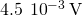Check Your Understanding (a) What is the magnetic flux through one turn of a solenoid of self-inductance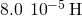when a current of 3.0 A flows through it? Assume that the solenoid has 1000 turns and is wound from wire of diameter 1.0 mm. (b) What is the cross-sectional area of the solenoid?

a.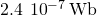; b.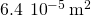### Summary

• Current changes in a device induce an emf in the device itself, called self-inductance,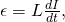where L is the self-inductance of the inductor and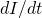is the rate of change of current through it. The minus sign indicates that emf opposes the change in current, as required by Lenz’s law. The unit of self-inductance and inductance is the henry (H), where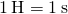.

• The self-inductance of a solenoid is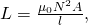where N is its number of turns in the solenoid, A is its cross-sectional area, l is its length, andis the permeability of free space.

• The self-inductance of a toroid is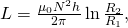where N is its number of turns in the toroid,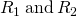are the inner and outer radii of the toroid, h is the height of the toroid, andis the permeability of free space.

### Conceptual Questions

Does self-inductance depend on the value of the magnetic flux? Does it depend on the current through the wire? Correlate your answers with the equationSelf-inductance is proportional to the magnetic flux and inversely proportional to the current. However, since the magnetic flux depends on the current I, these effects cancel out. This means that the self-inductance does not depend on the current. If the emf is induced across an element, it does depend on how the current changes with time.

Would the self-inductance of a 1.0 m long, tightly wound solenoid differ from the self-inductance per meter of an infinite, but otherwise identical, solenoid?

Discuss how you might determine the self-inductance per unit length of a long, straight wire.

Consider the ends of a wire a part of an RL circuit and determine the self-inductance from this circuit.

The self-inductance of a coil is zero if there is no current passing through the windings. True or false?

How does the self-inductance per unit length near the center of a solenoid (away from the ends) compare with its value near the end of the solenoid?

The magnetic field will flare out at the end of the solenoid so there is less flux through the last turn than through the middle of the solenoid.

### Problems

An emf of 0.40 V is induced across a coil when the current through it changes uniformly from 0.10 to 0.60 A in 0.30 s. What is the self-inductance of the coil?

0.24 H

The current shown in part (a) below is increasing, whereas that shown in part (b) is decreasing. In each case, determine which end of the inductor is at the higher potential.What is the rate at which the current though a 0.30-H coil is changing if an emf of 0.12 V is induced across the coil?

0.4 A/s

When a camera uses a flash, a fully charged capacitor discharges through an inductor. In what time must the 0.100-A current through a 2.00-mH inductor be switched on or off to induce a 500-V emf?

A coil with a self-inductance of 2.0 H carries a current that varies with time according to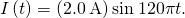Find an expression for the emf induced in the coil.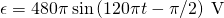A solenoid 50 cm long is wound with 500 turns of wire. The cross-sectional area of the coil is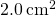What is the self-inductance of the solenoid?

A coil with a self-inductance of 3.0 H carries a current that decreases at a uniform rate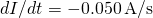. What is the emf induced in the coil? Describe the polarity of the induced emf.

0.15 V. This is the same polarity as the emf driving the current.

The current I(t) through a 5.0-mH inductor varies with time, as shown below. The resistance of the inductor is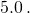Calculate the voltage across the inductor at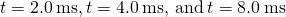.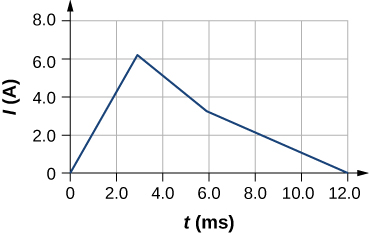A long, cylindrical solenoid with 100 turns per centimeter has a radius of 1.5 cm. (a) Neglecting end effects, what is the self-inductance per unit length of the solenoid? (b) If the current through the solenoid changes at the rate 5.0 A/s, what is the emf induced per unit length?

a. 0.089 H/m; b. 0.44 V/m

Suppose that a rectangular toroid has 2000 windings and a self-inductance of 0.040 H. If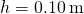, what is the ratio of its outer radius to its inner radius?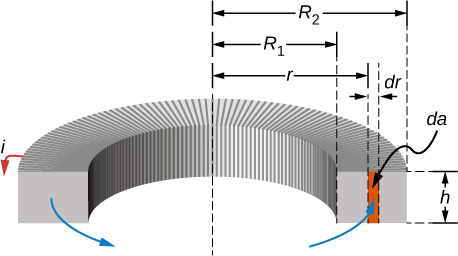What is the self-inductance per meter of a coaxial cable whose inner radius is 0.50 mm and whose outer radius is 4.00 mm?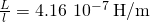### Glossary

inductor
part of an electrical circuit to provide self-inductance, which is symbolized by a coil of wire
self-inductance
effect of the device inducing emf in itself